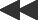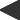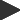basis: popcorn-fractal The orbits in 2 dimensions defined by x(0) = xpixel, y(0) = ypixel; x(n+1) = x(n) - h*sin(y(n) + tan(3*y(n)) y(n+1) = y(n) - h*sin(x(n) + tan(3*x(n)) are plotted for each screen pixel. One parameter: step size h. These types have been explored by Clifford A. Pickover, of the IBM Thomas J. Watson Research Center.They are regular Mandelbrot/Julia set pairs that may be plotted with or without the "biomorph" option Pickover used to create organic-looking beasties (see below). These types are produced with formulas built from the functions zz, zn, sin(z), and ez for complex z. Other values of the exponent give still other fractals.